# APPAREL

56 products found
Filtres

Gender

Size

Price

€10.00 - €170.00

Couleurs

Sport• S
• M
• L
• XL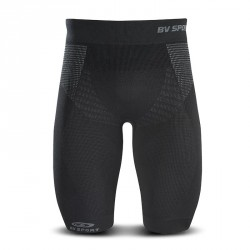-€20.00
• S
• M
• L
• XL-€26.00
• S
• M
• L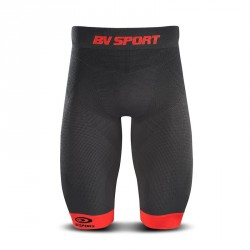-€26.00
• M
• L• S
• M
• M+
• L
• L+
• XL• S
• M
• M+
• L• S
• M
• M+
• L
• L+
• XL• S
• M
• M+
• L
• L+
• XL• S
• M
• L
• XL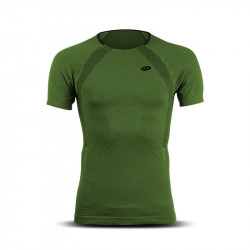• S• S
• L• S
• M
• L
• XL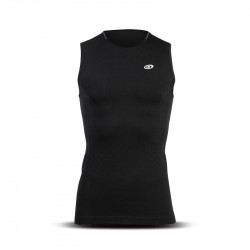• S
• XL• S
• L
• XL• S
• M
• L
• XL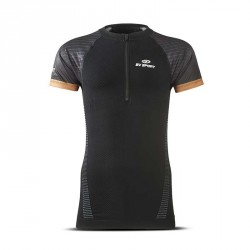• S
• XL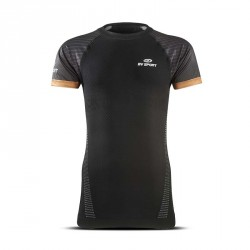-€20.00
• S-€24.95
• S
• M
• L-40%
• S• S
• M
• L
• XL• S
• M
• L
• XL• S
• M
• L• S
• M
• L• S
• M
• L• S
• M
• L
• XL• S
• M
• L
• XL• S
• M
• L• S
• M
• L• S
• M
• L-€11.95
• L
• XL-€11.95
• M
• L-€11.95
• L-€11.95
• S
• M
• L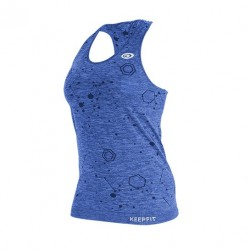-€11.95
• L-€11.95
• L-€11.95
• M
• L